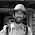## Sunday, December 16, 2012

### Weekend puzzle -- Count the Squares

I really like this one. The solution is easy and requires only basic math and good pattern recognition. If you don't spot the pattern, however, you've got a slog ahead of you.

There are hundreds of squares in this picture with dimensions ranging from 1x1 to 12x12. Exactly how many squares are there?

I've got a more detailed pedagogical discussion of the problem at You Do the Math. I'll post the answer in the comments section of this post later in the week.

1.The answer would hinge on whether all the squares must be used (144) or whether the answer could include any outline. The former can be done with arithmetic; the latter is a graduate study in topology if you add direction.

1.That's what I get for writing for a math crowd.

2.The answer is 1^2 + 2^2 + 3^2 + ... + 12^2. I go into a bit more detail on why this works at You Do the Math

3.This comment has been removed by the author.